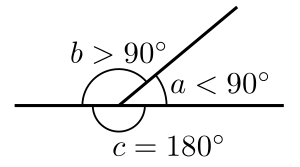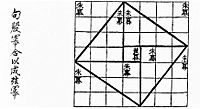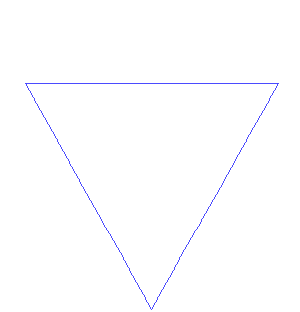# Geometry facts for kids

Kids Encyclopedia Facts
(Redirected from Geometric)

Geometry is a kind of mathematics that studies the size, shapes, and positions of things. There are flat (2D) shapes and solid (3D) shapes in geometry. Squares, circles and triangles are some of the simplest shapes in flat geometry. Cubes, cylinders, cones and spheres are simple shapes in solid geometry.

## Uses

Geometry can be used to measure the area and perimeter of a flat shape. It can also be used to measure a solid shape's volume and surface area.

Geometry can be used to calculate the size and shape of many things. For example, geometry can help people find:

## Origins

Geometry began as the art of measuring the shape of land so that it could be shared fairly between people. The word "geometry" means "to measure the land". It has grown from this to become one of the most important parts of mathematics. The Greek mathematician Euclid wrote the first book about geometry. Geometry is one of the oldest branches of mathematics.

## Examples

Geometry starts with a few simple ideas that are thought to be true, called axioms. Such as:

• A straight line is the shortest distance between two points. For example, Sophie pulls a piece of string from one point to another point. A straight line between the two points will follow the path of the tight string.
• A plane is a flat surface that does not stop in any direction. For example imagine a wall that extends in all directions infinitely.

## Important concepts in geometry

The following are some of the most important concepts in geometry.

### Axioms

Euclid took an abstract approach to geometry in his Elements, one of the most influential books ever written. Euclid introduced certain axioms, or postulates, expressing primary or self-evident properties of points, lines, and planes. He proceeded to rigorously deduce other properties by mathematical reasoning. The characteristic feature of Euclid's approach to geometry was its rigor, and it has come to be known as axiomatic or synthetic geometry. At the start of the 19th century, the discovery of non-Euclidean geometries by Nikolai Ivanovich Lobachevsky (1792–1856), János Bolyai (1802–1860), Carl Friedrich Gauss (1777–1855) and others led to a revival of interest in this discipline, and in the 20th century, David Hilbert (1862–1943) employed axiomatic reasoning in an attempt to provide a modern foundation of geometry.

### Points

Points are considered fundamental objects in Euclidean geometry. They have been defined in a variety of ways, including Euclid's definition as 'that which has no part' and through the use of algebra or nested sets. In many areas of geometry, such as analytic geometry, differential geometry, and topology, all objects are considered to be built up from points. However, there has been some study of geometry without reference to points.

### Lines

Euclid described a line as "breadthless length" which "lies equally with respect to the points on itself". In modern mathematics, given the multitude of geometries, the concept of a line is closely tied to the way the geometry is described. For instance, in analytic geometry, a line in the plane is often defined as the set of points whose coordinates satisfy a given linear equation, but in a more abstract setting, such as incidence geometry, a line may be an independent object, distinct from the set of points which lie on it. In differential geometry, a geodesic is a generalization of the notion of a line to curved spaces.

### Planes

A plane is a flat, two-dimensional surface that extends infinitely far. Planes are used in every area of geometry. For instance, planes can be studied as a topological surface without reference to distances or angles; it can be studied as an affine space, where collinearity and ratios can be studied but not distances; it can be studied as the complex plane using techniques of complex analysis; and so on.

### Angles

Euclid defines a plane angle as the inclination to each other, in a plane, of two lines which meet each other, and do not lie straight with respect to each other. In modern terms, an angle is the figure formed by two rays, called the sides of the angle, sharing a common endpoint, called the vertex of the angle.Acute (a), obtuse (b), and straight (c) angles. The acute and obtuse angles are also known as oblique angles.

In Euclidean geometry, angles are used to study polygons and triangles, as well as forming an object of study in their own right. The study of the angles of a triangle or of angles in a unit circle forms the basis of trigonometry.

In differential geometry and calculus, the angles between plane curves or space curves or surfaces can be calculated using the derivative.

### Curves

A curve is a 1-dimensional object that may be straight (like a line) or not; curves in 2-dimensional space are called plane curves and those in 3-dimensional space are called space curves.

In topology, a curve is defined by a function from an interval of the real numbers to another space. In differential geometry, the same definition is used, but the defining function is required to be differentiable Algebraic geometry studies algebraic curves, which are defined as algebraic varieties of dimension one.

### SurfacesA sphere is a surface that can be defined parametrically (by x = r sin θ cos φ, y = r sin θ sin φ, z = r cos θ) or implicitly (by x2 + y2 + z2r2 = 0.)

A surface is a two-dimensional object, such as a sphere or paraboloid. In differential geometry and topology, surfaces are described by two-dimensional 'patches' (or neighborhoods) that are assembled by diffeomorphisms or homeomorphisms, respectively. In algebraic geometry, surfaces are described by polynomial equations.

### Manifolds

A manifold is a generalization of the concepts of curve and surface. In topology, a manifold is a topological space where every point has a neighborhood that is homeomorphic to Euclidean space. In differential geometry, a differentiable manifold is a space where each neighborhood is diffeomorphic to Euclidean space.

Manifolds are used extensively in physics, including in general relativity and string theory

### Topologies and metricsVisual checking of the Pythagorean theorem for the (3, 4, 5) triangle as in the Zhoubi Suanjing 500–200 BC. The Pythagorean theorem is a consequence of the Euclidean metric.

A topology is a mathematical structure on a set that tells how elements of the set relate spatially to each other. The best-known examples of topologies come from metrics, which are ways of measuring distances between points. For instance, the Euclidean metric measures the distance between points in the Euclidean plane, while the hyperbolic metric measures the distance in the hyperbolic plane. Other important examples of metrics include the Lorentz metric of special relativity and the semi-Riemannian metrics of general relativity.

### Compass and straightedge constructions

Classical geometers paid special attention to constructing geometric objects that had been described in some other way. Classically, the only instruments allowed in geometric constructions are the compass and straightedge. Also, every construction had to be complete in a finite number of steps. However, some problems turned out to be difficult or impossible to solve by these means alone, and ingenious constructions using parabolas and other curves, as well as mechanical devices, were found.

### DimensionThe Koch snowflake, with fractal dimension=log4/log3 and topological dimension=1

Where the traditional geometry allowed dimensions 1 (a line), 2 (a plane) and 3 (our ambient world conceived of as three-dimensional space), mathematicians have used higher dimensions for nearly two centuries. Dimension has gone through stages of being any natural number n, possibly infinite with the introduction of Hilbert space, and any positive real number in fractal geometry. Dimension theory is a technical area, initially within general topology, that discusses definitions; in common with most mathematical ideas, dimension is now defined rather than an intuition. Connected topological manifolds have a well-defined dimension; this is a theorem (invariance of domain) rather than anything a priori.

The issue of dimension still matters to geometry, in the absence of complete answers to classic questions. Dimensions 3 of space and 4 of space-time are special cases in geometric topology. Dimension 10 or 11 is a key number in string theory. Research may bring a satisfactory geometric reason for the significance of 10 and 11 dimensions.

### Symmetry

The theme of symmetry in geometry is nearly as old as the science of geometry itself. Symmetric shapes such as the circle, regular polygons and platonic solids held deep significance for many ancient philosophers and were investigated in detail before the time of Euclid. Symmetric patterns occur in nature and were artistically rendered in a multitude of forms, including the graphics of M. C. Escher. Nonetheless, it was not until the second half of 19th century that the unifying role of symmetry in foundations of geometry was recognized. Felix Klein's Erlangen program proclaimed that, in a very precise sense, symmetry, expressed via the notion of a transformation group, determines what geometry is. Symmetry in classical Euclidean geometry is represented by congruences and rigid motions, whereas in projective geometry an analogous role is played by collineations, geometric transformations that take straight lines into straight lines. However it was in the new geometries of Bolyai and Lobachevsky, Riemann, Clifford and Klein, and Sophus Lie that Klein's idea to 'define a geometry via its symmetry group' proved most influential. Both discrete and continuous symmetries play prominent roles in geometry, the former in topology and geometric group theory, the latter in Lie theory and Riemannian geometry.

A different type of symmetry is the principle of duality in projective geometry (see Duality (projective geometry)) among other fields. This meta-phenomenon can roughly be described as follows: in any theorem, exchange point with plane, join with meet, lies in with contains, and you will get an equally true theorem. A similar and closely related form of duality exists between a vector space and its dual space.

### Non-Euclidean geometry

In the nearly two thousand years since Euclid, while the range of geometrical questions asked and answered inevitably expanded, the basic understanding of space remained essentially the same. Immanuel Kant argued that there is only one, absolute, geometry, which is known to be true a priori by an inner faculty of mind: Euclidean geometry was synthetic a priori. This dominant view was overturned by the revolutionary discovery of non-Euclidean geometry in the works of Bolyai, Lobachevsky, and Gauss (who never published his theory). They demonstrated that ordinary Euclidean space is only one possibility for development of geometry. A broad vision of the subject of geometry was then expressed by Riemann in his 1867 inauguration lecture Über die Hypothesen, welche der Geometrie zu Grunde liegen (On the hypotheses on which geometry is based), published only after his death. Riemann's new idea of space proved crucial in Einstein's general relativity theory, and Riemannian geometry, that considers very general spaces in which the notion of length is defined, is a mainstay of modern geometry.

## Related pagesGeometry Facts for Kids. Kiddle Encyclopedia.